Courses

# Residual Property Relations Civil Engineering (CE) Notes | EduRev

## Civil Engineering (CE) : Residual Property Relations Civil Engineering (CE) Notes | EduRev

The document Residual Property Relations Civil Engineering (CE) Notes | EduRev is a part of the Civil Engineering (CE) Course Thermodynamics.
All you need of Civil Engineering (CE) at this link: Civil Engineering (CE)

Residual Property Relations

An alternate method of computing energy and entropy changes for real gases involves the definition of residual property. The specific residual property M R is defined as follows:

MR = M (T,P) − Mig (T,P)                ...(5.24)

Where, M (T, P) is the specific property of a real gas at a given T & P, and Mig (T,P) is the value of the same property if the gas were to behave ideally at the same T & P. Thus for example:
V= V −Vig

Using the generalized compressibility factor ‘Z’:

VR = (ZRT / P) − ( RT / P)

VR = (Z − 1) RT / P                ...(5.25)

The residual properties are usually used for gases only. Using such a property for a liquid (or solid) is inconvenient as then it would also include the property change of vapourization (and solidification) which generally are large in magnitude. This detracts from the advantage of working with the residual property as a measure of small corrections to ideal gas behaviour. Thus the use of residual functions is restricted to prediction of real gas behaviour only. To exploit the concept of residual properties we take advantage of the Gibbs free energy as it can be used as a generating function for other thermodynamic properties.

Derivation of Residual functions:

We start from the generic equation: G = H − TS                 ...(5.2)

And dG = VdP − SdT                          ...(5.7)

Taking the total differential for the function G / RT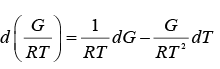...(5.26)

Substituting eqn. 5.2 and 5.7 in 5.26 we get: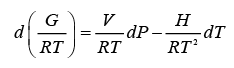...(5.27)

One may write the same equation specifically for an ideal gas, whence: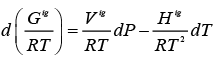...(5.28)

Subtracting eqn. 5.28 from 5.27: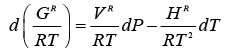...(5.30)

Thus we may write the following further generative relations: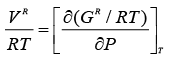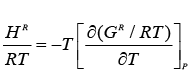...(5.31)

And further: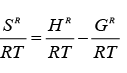...(5.32)

From eqn. 5.29: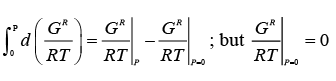Thus: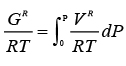...(5.33)

Putting eqn. 5.25 in 5.33: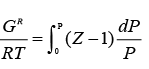...(5.34)

Differentiating eqn. 5.34 w.r.t T in accordance with 5.31 gives: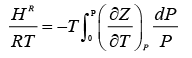...(5.35)

Finally using eqns 5.32, 5.34, and 5.35: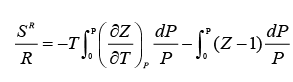...(5.36)

The last two equations may be expressed in alternative forms in terms of reduced temperature and pressure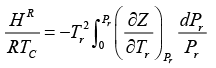...(5.37)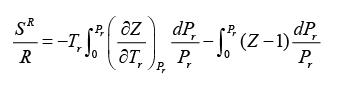...(5.38)

Offer running on EduRev: Apply code STAYHOME200 to get INR 200 off on our premium plan EduRev Infinity!

## Thermodynamics

56 videos|92 docs|33 tests

,

,

,

,

,

,

,

,

,

,

,

,

,

,

,

,

,

,

,

,

,

;